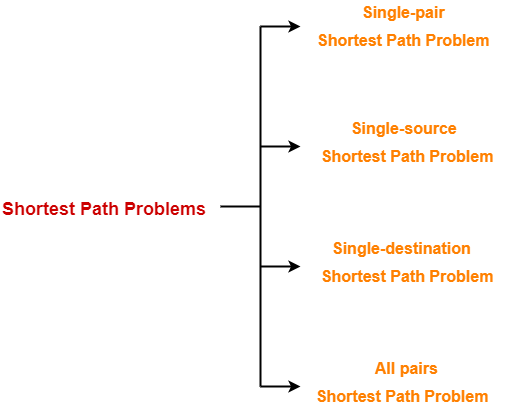# Shortest Path Problem | Shortest Path Algorithms | Examples

## Shortest Path Problem-

In data structures,

• Shortest path problem is a problem of finding the shortest path(s) between vertices of a given graph.
• Shortest path between two vertices is a path that has the least cost as compared to all other existing paths.

## Shortest Path Algorithms-

Shortest path algorithms are a family of algorithms used for solving the shortest path problem.

### Applications-

Shortest path algorithms have a wide range of applications such as in-

• Logistics Research

## Types of Shortest Path Problem-

Various types of shortest path problem are-1. Single-pair shortest path problem
2. Single-source shortest path problem
3. Single-destination shortest path problem
4. All pairs shortest path problem

## Single-Pair Shortest Path Problem-

• It is a shortest path problem where the shortest path between a given pair of vertices is computed.
• A* Search Algorithm is a famous algorithm used for solving single-pair shortest path problem.

## Single-Source Shortest Path Problem-

• It is a shortest path problem where the shortest path from a given source vertex to all other remaining vertices is computed.
• Dijkstra’s Algorithm and Bellman Ford Algorithm are the famous algorithms used for solving single-source shortest path problem.

## Single-Destination Shortest Path Problem-

• It is a shortest path problem where the shortest path from all the vertices to a single destination vertex is computed.
• By reversing the direction of each edge in the graph, this problem reduces to single-source shortest path problem.
• Dijkstra’s Algorithm is a famous algorithm adapted for solving single-destination shortest path problem.

## All Pairs Shortest Path Problem-

• It is a shortest path problem where the shortest path between every pair of vertices is computed.
• Floyd-Warshall Algorithm and Johnson’s Algorithm are the famous algorithms used for solving All pairs shortest path problem.

Next Article- Dijkstra’s Algorithm

Get more notes and other study material of Design and Analysis of Algorithms.

Watch video lectures by visiting our YouTube channel LearnVidFun.

SummaryArticle Name
Shortest Path Problem | Shortest Path Algorithms | Examples
Description
Shortest Path Problem in Data Structure is a problem of finding the shortest path between vertices of a given graph. Shortest Path Algorithms are a family of algorithms used for solving Shortest Path Problem. Examples.
Author
Publisher Name
Gate Vidyalay
Publisher Logo